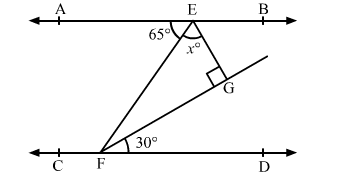# In the given figure, AB || CD and EF is a transversal. If ∠AEF = 65°, ∠DFG = 30°,

Question:

In the given figure, AB || CD and EF is a transversal. If ∠AEF = 65°, ∠DFG = 30°, ∠EGF = 90° and ∠GEF = x°, find the value of x.Solution:

It is given that, AB || CD and EF is a transversal.

∴ ∠EFD = ∠AEF          (Pair of alternate angles)

⇒ ∠EFD = 65°

⇒ ∠EFG + ∠GFD = 65°

⇒ ∠EFG + 30° = 65°

⇒ ∠EFG = 65° − 30° = 35°

In ∆EFG,

∠EFG + ∠GEF + ∠EGF = 180°           (Angle sum property)

⇒ 35° + x + 90° = 180°

⇒ 125° + x = 180°

⇒ x = 180° − 125° = 55°

Thus, the value of x is 55°.